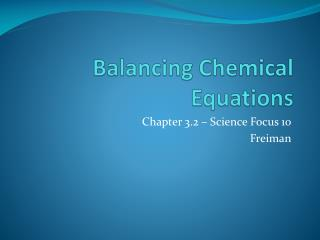DownloadDownload PresentationBalancing Chemical Equations

# Balancing Chemical Equations

Télécharger la présentation## Balancing Chemical Equations

- - - - - - - - - - - - - - - - - - - - - - - - - - - E N D - - - - - - - - - - - - - - - - - - - - - - - - - - -
##### Presentation Transcript

1. Balancing Chemical Equations Chapter 3.2 – Science Focus 10 Freiman

2. Chemical Equations Basics C3H8(g) + 5O2(g) 3CO2(g) + 4H2O(g) Reactants Products C3H8(g) + 5O2(g) 3CO2(g) + 4H2O(g) Number of carbons in the compound Number of CO2 molecules in balanced equation

3. Balancing Equations • The Law of Conservation of Mass states: • MASS OF REACTANTS = MASS OF PRODUCTS • Formula equations must have the same number of each atom on the reactant and product side of the equation. • Ex) N2 + H2  NH3 • Nitrogens and hydrogens are NOT balanced!

4. Steps to Balancing Equations • 1. Write out the unbalanced equation (this may be given to you already) • 2. Find an unbalanced element and multiply the corresponding compounds on both sides of the equation until that element is balanced • 3. Repeat for all other elements

5. Tip • 1. Leave single or molecular elements until the end.

6. Examples • On board • (2nd day – do harder ones – DOUBLING and Polyatomics that break apart)